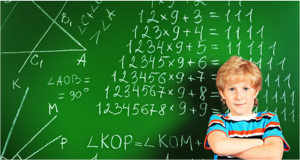Vedic Mathematics is the name given to the ancient system of Indian Mathematics which was rediscovered from the Vedas. According to this research all of mathematics is based on sixteen Sutras, or word-formulae. For example, ‘Vertically and crosswise` is one of these Sutras. These formulae describe the way the mind naturally works and are therefore a great help in directing the student to the appropriate method of solution. In the Vedic system ‘difficult’ problems or huge sums can often be solved immediately by the Vedic method. These striking and beautiful methods are just a part of a complete system of mathematics which is far more systematic than the modern ‘system’. The simplicity of Vedic Mathematics means that calculations can be carried out mentally (though the methods can also be written down). There are many advantages in using a flexible, mental system.

What is the importance of Vedic Math’s in today’s World- Mathematics is the body of knowledge centered on concepts such as quantity, structure, space, and change, and also the academic discipline which studies them. Vedic Math’s is the basis for all modern concepts of math’s so it is the backbone even in astrology and scientific calculations. Well, it gives you some short cut mantras to perform processes like long division, complex multiplication, finding square roots, cube roots etc.

Vedic Math’s is more than just a few shortcuts; it is actually another way of solving problems. U can actually get into deeper concepts like calculus using this. Ancient Mathematicians discovered the Pythagoras theorem and rudimentary calculus ages before the western world even thought of it. It is actually an alternate way of solving the problem.

Schools Of Excellence conduct exam of each level and provide certificate.

### Benefits of Vedic Math’s Learning

• Better marks in Mathematics
• Better marks in other subjects
• Improved Self Confidence
• Better Concentration
• Mental Arithmetic skill
• Fast Calculations
• Better Flexibility
• Better Creativity
• Improved Memory